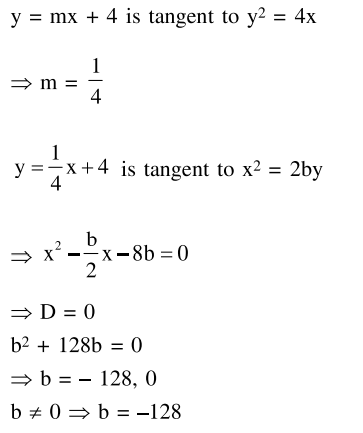# If y=m x+4 is a tangent to both the parabolas,`
Question:

If $y=m x+4$ is a tangent to both the parabolas, $y^{2}=4 x$ and $x^{2}=2 b y$, then $b$ is equal to :

1. 128

2. $-64$

3. $-128$

4. $-32$

Correct Option: , 3

Solution: